• Overfitting how to detect 1、train/evalute /test splitting batchsz = 128 (x, y), (x_test, y_test) = datasets.mnist.load_data() print('datasets:', x.shape, y.shape, x.min(), x.max()) ...
Overfitting
how to detect
1、train/evalute /test splitting
batchsz = 128
(x, y), (x_test, y_test) = datasets.mnist.load_data()
print('datasets:', x.shape, y.shape, x.min(), x.max())

idx = tf.range(60000)
idx = tf.random.shuffle(idx)
x_train, y_train = tf.gather(x, idx[:50000]), tf.gather(y, idx[:50000])
x_val, y_val = tf.gather(x, idx[-10000:]) , tf.gather(y, idx[-10000:])
print(x_train.shape, y_train.shape, x_val.shape, y_val.shape)
db_train = tf.data.Dataset.from_tensor_slices((x_train,y_train))
db_train = db_train.map(preprocess).shuffle(50000).batch(batchsz)

db_val = tf.data.Dataset.from_tensor_slices((x_val,y_val))
db_val = db_val.map(preprocess).shuffle(10000).batch(batchsz)

2、k_fold cross-validation
Reduce Overfitting
More data
3、constrain model complexity
shallow
奥卡姆剃刀原理
regularization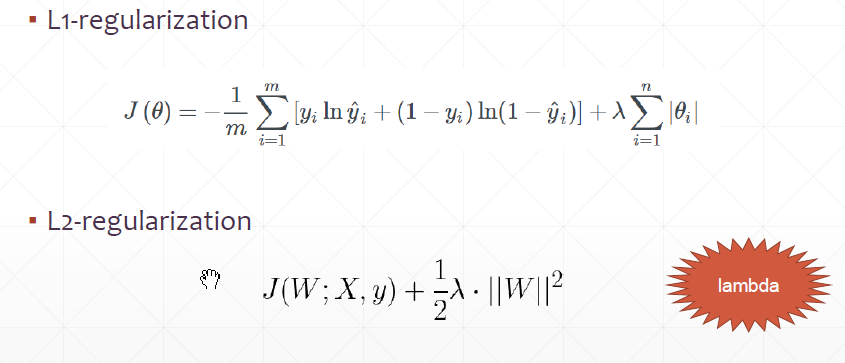手动添加


for step, (x,y) in enumerate(db):

# [b, 28, 28] => [b, 784]
x = tf.reshape(x, (-1, 28*28))
# [b, 784] => [b, 10]
out = network(x)
# [b] => [b, 10]
y_onehot = tf.one_hot(y, depth=10)
# [b]
loss = tf.reduce_mean(tf.losses.categorical_crossentropy(y_onehot, out, from_logits=True))

loss_regularization = []
for p in network.trainable_variables:
loss_regularization.append(tf.nn.l2_loss(p))
loss_regularization = tf.reduce_sum(tf.stack(loss_regularization))
loss = loss + 0.0001 * loss_regularization

if step % 100 == 0:

print(step, 'loss:', float(loss), 'loss_regularization:', float(loss_regularization))


自动添加
keras.models.Sequential([
keras.layers.Dense(16,kernel_regularizer=keras.regularizers.l2(0.0001)
,activation=tf.nn.relu,input_shape=())
])

tricks
momentum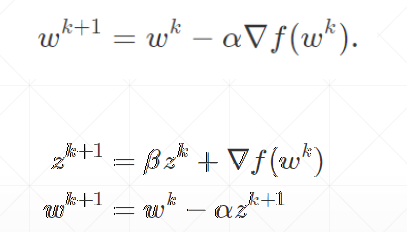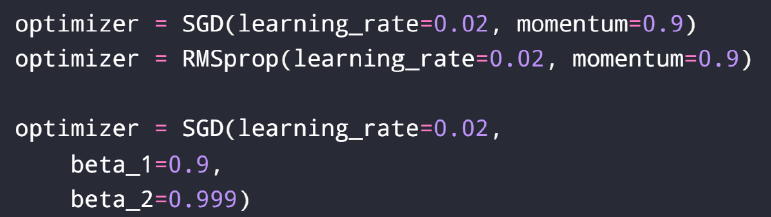learning rate decay
4、dropout
network = Sequential([layers.Dense(256, activation='relu'),
layers.Dropout(0.5), # 0.5 rate to drop
layers.Dense(128, activation='relu'),
layers.Dropout(0.5), # 0.5 rate to drop
layers.Dense(64, activation='relu'),
layers.Dense(32, activation='relu'),
layers.Dense(10)])
network.build(input_shape=(None, 28*28))
network.summary()

5、early stopping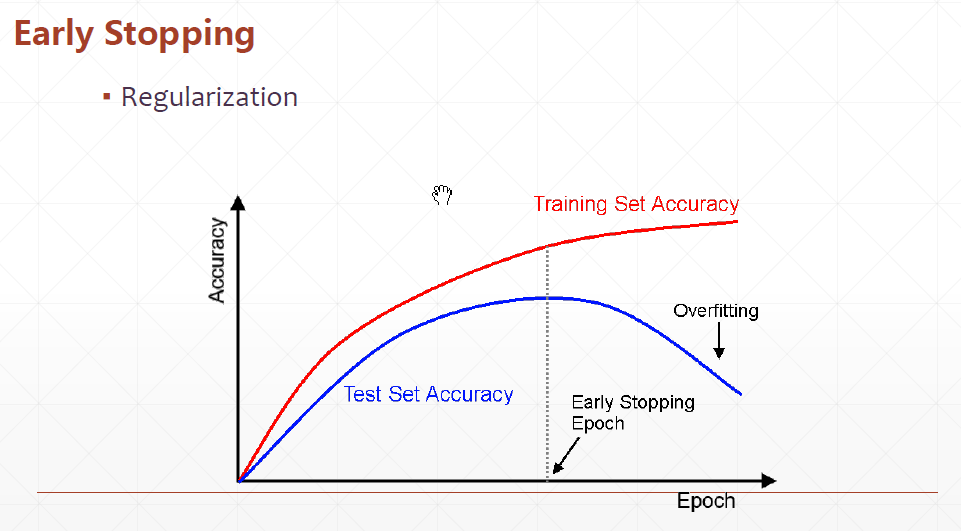validation set to select patametersMonitor validation performancestop at the highest val perf

展开全文tensorflow 过拟合 过拟合解决方法
• 深度学习神经网络欠拟合到拟合的调整方法及示例代码 1. 深度神经网络结构调整说明 当深度神经网络可以对少量样本进行很好的拟合，而对较多的样本无法很好拟合的时候，很可能是深度网络的层数不够深，即深度网络...
深度学习神经网络从欠拟合到拟合的调整方法及示例代码
1.     深度神经网络结构调整说明
当深度神经网络可以对少量样本进行很好的拟合，而对较多的样本无法很好拟合的时候，很可能是深度网络的层数不够深，即深度网络的特征无法很好地表征所有样本的特征。此时，可以通过加深网络的层次来使得深度网络对较多的样本进行拟合。
另外，深度网络对于分类样本的拟合能力强于对于连续值样本的拟合能力。即同样的深度网络如果能拟合10组分类的样本，可能只能拟合3组连续值的样本。分类样本采用softmaxwithloss作为损失函数，连续值样本采用欧式距离损失函数。以下例子已连续值样本作为拟合的方式。
2.     少样本合适拟合的示例代码
本例包含四组样本，深度网络较好地拟合。网络参数为：
netsize=[inputsize,5,6,8,7,5,4];
(1)   Main_function
clear all
clc
close all
TrainData =[1   2 3   4   5  6  7   8 9  10
1   9  17 25  33  41  49  57 65  73];
batchsize=4;
TrainData=TrainData(:,1:batchsize);
TrainLabel=[1    0.3  0.8  0.2;
1.8  0.8   1.2 1.1];
classnum=2;%输出端数目

%获取数据的维度
inputsize=size(TrainData ,1);
%获取数据的数量
datanum=size(TrainData ,2);
% 用一个向量来定义网络的深度，以及每层神经元数目。
netsize=[inputsize,5,6,8,7,5,4];
% netsize=[inputsize,5,6,8,9,9,8,7,5,4];

%网络最后一层神经元数数目，再考虑一个偏置。
lastsize=netsize(end)+1;

%初始化网络参数，以结构体的形式保存。
stack = initializeNet(netsize);

% 在训练时，往往需要将参数转成一列向量，提供给损失函数。
% stack ->stackTheta，netconfig保存一些结构参数。
[stackTheta, netconfig] =stack2params(stack);

% 指定固定的最后一层的初始化的值;
rand('state',2)
lastTheta = 0.0005 * randn(lastsize *classnum, 1);

%最终网络需要的参数
Theta=[ lastTheta ; stackTheta ];
%lastTheta表示深度网络最后一层的权值

% the following part is for the traingepoch.
% batchsize=5;
%%每次训练的小批量样本数</span>
batchnum=floor(size(TrainData,2)/batchsize);
DataNum=size(TrainData,2);
alpha=1e-2;
%这是学习率，一般随着网络的悬念都需要不断的减小
lambda = 1e-4; % Weight decay parameter
for epoch=1:16000
v_epoch=epoch
if epoch>=16000
alpha=1e-3;
end
idx=randperm(DataNum);
for t=1:batchnum
subdata=TrainData(:,idx((t-1)*batchsize+1:(t)*batchsize));
sublabel=TrainLabel(:,idx((t-1)*batchsize+1:(t)*batchsize));
end
end
plot(log(cost))

(2)   drelu
function dre= drelu(x)
dre=zeros(size(x));
dre(x>0)=1;
dre(x==0)=0.5; %这句可以不要
end
(3)   relu
function re = relu(x)
re = max(x,0)-1;
end
(4)   params2stack
function stack = params2stack(params, netconfig)
depth =numel(netconfig.layersizes);
stack = cell(depth,1);
prevLayerSize =netconfig.inputsize;  %the size of the previous layer
curPos = double(1); % mark current position in parametervector
for d = 1:depth
% Create layer d
stack{d} = struct;
% Extract weights
wlen =double(netconfig.layersizes{d} * prevLayerSize);
stack{d}.w =reshape(params(curPos:curPos+wlen-1), netconfig.layersizes{d}, prevLayerSize);
curPos = curPos+wlen;
% Extract bias
blen =double(netconfig.layersizes{d});
stack{d}.b =reshape(params(curPos:curPos+blen-1), netconfig.layersizes{d}, 1);
curPos = curPos+blen;
% Set previous layer size
prevLayerSize =netconfig.layersizes{d};
end
end
(5)   ReLUDNNCost
%参数获取的一些操作
lastTheta =reshape(theta(1:lasthiddenSize*numClasses), numClasses, lasthiddenSize);
%从theta向量中抽取网络权值参数并转化
stack =params2stack(theta(lasthiddenSize*numClasses+1:end), netconfig);

%这里保存在应用BP算法求梯度时需要的数据
PARA=cell(numel(stack),1);
%传进来的样本数
datanum=size(trainData,2);

%开始前馈，网络虽然多层，但只是重复而已
data=trainData;
for d = 1:numel(stack)
PARA{d}.a=data;
z2=(stack{d}.w*data)+stack{d}.b*ones(1,datanum);
%ReLU函数
a2=relu(z2);
data=a2;
%ReLU函数的导函数
PARA{d}.daz=drelu(z2);
end
a2=[a2;ones(1,datanum)];
%开始求解损失
groundTruth=trainLabels;
v_groundTruth=groundTruth
M = lastTheta*a2;
v_M=M

% 损失函数，
cost=sum(sum((groundTruth-M).^2))./datanum;
%最后一层神经元的目标函数对lastTheta 的导数，
% 输出层误差传导至倒数第二层神经元的值
predelta=-lastTheta'*(groundTruth-M);

predelta=predelta(1:end-1,:);
for d = numel(stack):-1:1
delta=predelta.*PARA{d}.daz;
predelta=stack{d}.w'*delta;
end
end
(6)   stack2params
function [params, netconfig] = stack2params(stack)
params = [];
for d = 1:numel(stack)
params = [params ; stack{d}.w(:) ;
stack{d}.b(:) ];
end
if nargout > 1
if numel(stack) == 0
netconfig.inputsize = 0;
netconfig.layersizes = {};
else
netconfig.inputsize = size(stack{1}.w,2);
netconfig.layersizes = {};
for d =1:numel(stack)
netconfig.layersizes =[netconfig.layersizes ; size(stack{d}.w,1)];
end
end
end
end

(7)   initializeNet
function stack = initializeNet(netsize)
layersize=length(netsize(:));
stack = cell(layersize-1,1);
for l=1:layersize-1
hiddenSize=netsize(l+1);
visibleSize=netsize(l);
r =sqrt(6) /sqrt(hiddenSize+visibleSize+1);
rand('state',2)
stack{l}.w= rand(hiddenSize, visibleSize) *2 * r - r; stack{l}.b= zeros(hiddenSize, 1);
end
end

(8)   运行结果
深度网络收敛，运行结果如下图所示：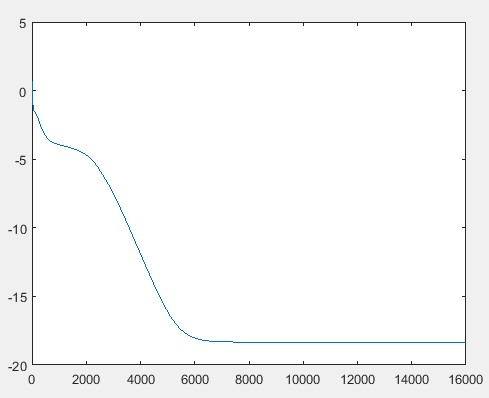(F1)
输出标签值如下：
v_groundTruth =
0.2000    0.3000    1.0000   0.8000
1.1000    0.8000    1.8000   1.2000
深度网络实际拟合值如下：
v_M =
0.2001    0.3001    0.9999   0.7999
1.0999    0.8000   1.7999    1.2000
由上面数据比较可见，两者差距较小，深度网络较好地拟合。

3.     多样本深度网络欠拟合代码
本例在第2部分中的四组样本的基础上，增加一组样本，深度网络无法拟合。网络参数为：
netsize=[inputsize,5,6,8,7,5,4];
(1)   Main_function
clear all
clc
close all
TrainData=[1   2 3   4   5  6  7   8 9  10
1  9  17  25 33  41 49  57 65 73];
batchsize=5;
TrainData=TrainData(:,1:batchsize);
TrainLabel=[1    0.3  0.8  0.2  0.67;
1.8 0.8   1.2  1.1 0.18];
classnum=2;%输出端数目

%获取数据的维度
inputsize=size(TrainData,1);
%获取数据的数量
datanum=size(TrainData,2);
% 用一个向量来定义网络的深度，以及每层神经元数目。
netsize=[inputsize,5,6,8,7,5,4];
% netsize=[inputsize,5,6,8,9,9,8,7,5,4];

%网络最后一层神经元数数目，再考虑一个偏置。
lastsize=netsize(end)+1;

%初始化网络参数，以结构体的形式保存。
stack =initializeNet(netsize);

% 在训练时，往往需要将参数转成一列向量，提供给损失函数。
% stack->stackTheta，netconfig保存一些结构参数。
[stackTheta,netconfig] = stack2params(stack);

% 指定固定的最后一层的初始化的值;
rand('state',2)
lastTheta =0.0005 * randn(lastsize * classnum, 1);

%最终网络需要的参数
Theta=[lastTheta ; stackTheta ];
%lastTheta表示深度网络最后一层的权值

% the followingpart is for the traing epoch.
% batchsize=5;
%%每次训练的小批量样本数</span>
batchnum=floor(size(TrainData,2)/batchsize);
DataNum=size(TrainData,2);
alpha=1e-2;
%这是学习率，一般随着网络的悬念都需要不断的减小
lambda = 1e-4; %Weight decay parameter
forepoch=1:16000
v_epoch=epoch
if epoch>=16000
alpha=1e-3;
end
idx=randperm(DataNum);
for t=1:batchnum
subdata=TrainData(:,idx((t-1)*batchsize+1:(t)*batchsize));
sublabel=TrainLabel(:,idx((t-1)*batchsize+1:(t)*batchsize));
end
end
plot(log(cost))

(2)   运行结果
深度网络无法很好地收敛，运行结果如下图所示：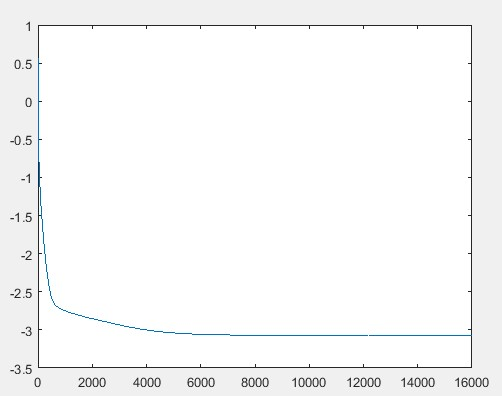(F2)
输出标签值如下：
v_groundTruth =
0.6700   0.3000    0.2000    1.0000   0.8000
0.1800    0.8000   1.1000    1.8000    1.2000
深度网络实际输出值如下
v_M =
0.6114    0.4340    0.4819   0.9996    0.4429
0.1749    0.8124   1.1275    1.8001    1.1651
由上面数据比较可见，两者差距较大，深度网络无法很好地拟合。
4.     加深网络后多样本可以很好地拟合
本例在第3部分中的五组样本，深度网络无法拟合的情况下，加深网络深度，网络参数为：
netsize=[inputsize,5,6,8,9,9,8,7,5,4]; 深度网络很好地拟合。

(1)   Main_function
clear all
clc
close all
TrainData =[1   2 3   4   5  6  7   8 9  10
1   9  17 25  33  41 49 57 65 73];
% TrainData=TrainData(:,1:6);
% TrainLabel=[1 0.3 1.8 0.87 0.66 0.83];
% batchsize=6;
% classnum=1;%输出端数目
batchsize=5;
TrainData=TrainData(:,1:batchsize);
TrainLabel=[1    0.3  0.8  0.2  0.67;
1.8  0.8   1.2 1.1  0.18];
% TrainLabel=[1    0.3  0.8  0.2;
%             1.8  0.8  1.2  1.1];
classnum=2;%输出端数目

%获取数据的维度
inputsize=size(TrainData ,1);
%获取数据的数量
datanum=size(TrainData ,2);
% 用一个向量来定义网络的深度，以及每层神经元数目。
netsize=[inputsize,5,6,8,9,9,8,7,5,4];

%网络最后一层神经元数数目，再考虑一个偏置。
lastsize=netsize(end)+1;

%初始化网络参数，以结构体的形式保存。
stack = initializeNet(netsize);

% 在训练时，往往需要将参数转成一列向量，提供给损失函数。
% stack ->stackTheta，netconfig保存一些结构参数。
[stackTheta, netconfig] =stack2params(stack);

% 指定固定的最后一层的初始化的值;
rand('state',2)
lastTheta = 0.0005 * randn(lastsize *classnum, 1);

%最终网络需要的参数
Theta=[ lastTheta ; stackTheta ];
%lastTheta表示深度网络最后一层的权值

% the following part is for the traing epoch.
% batchsize=5;
%%每次训练的小批量样本数</span>
batchnum=floor(size(TrainData,2)/batchsize);
DataNum=size(TrainData,2);
alpha=1e-2;
%这是学习率，一般随着网络的悬念都需要不断的减小
lambda = 1e-4; % Weight decay parameter
for epoch=1:16000
v_epoch=epoch
if epoch>=16000
alpha=1e-3;
end
idx=randperm(DataNum);
for t=1:batchnum
subdata=TrainData(:,idx((t-1)*batchsize+1:(t)*batchsize));
sublabel=TrainLabel(:,idx((t-1)*batchsize+1:(t)*batchsize));
end
end
plot(log(cost))

(2)   运行结果
深度网络很好地收敛，运行结果如下图所示：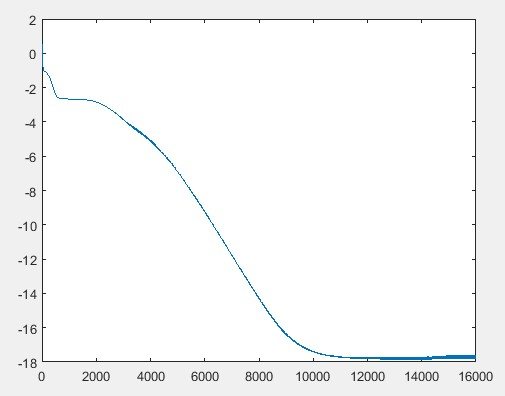(F3)
输出标签值如下：
v_groundTruth =
0.6700    0.3000    0.2000   1.0000    0.8000
0.1800    0.8000   1.1000    1.8000    1.2000
深度网络实际输出值如下：
v_M =
0.6699    0.3000   0.2002    0.9999    0.7999
0.1800    0.8000   1.0999    1.7998    1.2001
由上面数据比较可见，两者差距较小，深度网络很好地拟合。
5.     总结
从第2部分至第5部分的训练样本及运行结果可以看出，当深度网络较浅时，可能会面临多样本数据无法很好拟合的情况。此时，如果加深网络层数，则可能会使得网络很好地拟合多组训练样本。
展开全文深度学习 matlab
• 过拟合 (Overfitting)及其解决方法 欠拟合(梯度消失) 梯度消失： 根源在于激活函数，每次的求导，使得梯度的计算随着层数的增加而呈现指数级的递减趋势。离输出层越远，梯度减少的越明显。 而为了防止神经网络在...
 往期回顾
. 面试之深度学习中的过拟合问题 . 过拟合 (Overfitting)及其解决方法

欠拟合(梯度消失)
梯度消失： 根源在于激活函数，每次的求导，使得梯度的计算随着层数的增加而呈现指数级的递减趋势。离输出层越远，梯度减少的越明显。
而为了防止神经网络在训练过程中梯度消失的问题，一般采用合理的激活函数，如ReLU ，maxout来取代传统的sigmoid函数。
过拟合(梯度爆炸)
过拟合 一般指的是在模型选择的过程中，选择的模型参数过多，导致对训练数据的预测很好，但对未知数据的预测很差的现象。
在深度神经网络中，网络的层数更深，训练的样本往往更少的情况下，会使得过拟合现象更加严重。Dropout结合单模型和多模型的优点，能够很好地解决过拟合问题。
Dropout的思想 是每一层迭代的过程中，会随机让网络某些节点（神经元）不参与训练，同时把这些暂时丢弃的神经元的相关的所有边全部去掉，相应的权重不会在这一次迭代中更新，每次迭代训练都会重复这个操作。而丢掉的神经元只是暂时不更新，下一次迭代会重新参与随机化的Dropout。
展开全文深度学习
• 一、过拟合欠拟合及其解决方案 我们将探究模型训练中经常出现的两类典型问题： 一类是模型无法得到较低的训练误差，我们将这一现象...当神经网络的层数较多时，模型的数值稳定性容易变差。 假设一个层数为的多层感知
• 文章目录深度学习基础3一、过拟合欠拟合1.概念2.解决过拟合欠拟合的方法（1）权重缩减（2）丢弃法二、梯度消失与梯度爆炸1.消失与爆炸2.随机初始化3.影响模型效果的其他因素三、循环神经网络进阶1.门控循环神经网络/...
• 如网络太深，神经网络中；或者线性回归中模型的阶次 过多的变量特征 训练数据过少 如何解决过拟合： 降低模型复杂度 减少特征数目 增加数据 正则化等 欠拟合问题（high bias） 欠拟合：指模型太过简单，不能对训练...
• 一、过拟合 就是所建的机器学习模型或者是深度学习模型在训练样本中表现得过于优越，在验证数据集以及测试数据集中却表现不佳。...二、欠拟合 就是所建的模型在训练样本中的表现都很一般 可以通过增加网...
一、过拟合
就是所建的机器学习模型或者是深度学习模型在训练样本中表现得过于优越，在验证数据集以及测试数据集中却表现不佳。
导致这种现象的原因有以下2种：
①数据集特征单一，或者数据量过少
可以丰富数据集
②网络层数较深，参数过多
适当的减少网络层数，激活函数使用relu也是一种方法，因为他会将小于0的部分全部归为0，从而造成了网络的稀疏性，并且减少了参数的相互依存关系，缓解了过拟合问题的发生

二、欠拟合
就是所建的模型在训练样本中的表现都很一般
可以通过增加网络复杂度或者在模型中增加多点特征点

三、激活函数
作用：通过激活函数，神经网络就可以拟合各种非线性曲线，所以激活函数肯定都是非线性的
① sigmod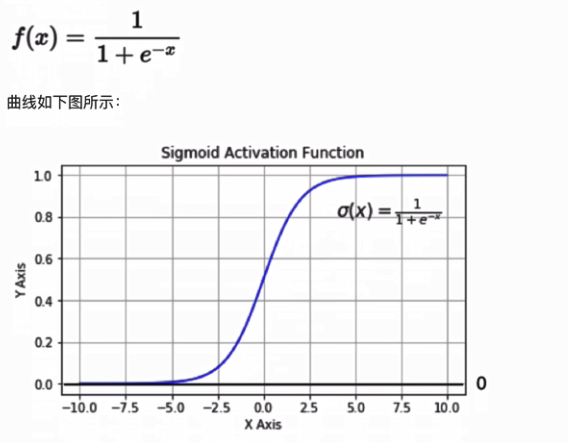②tanh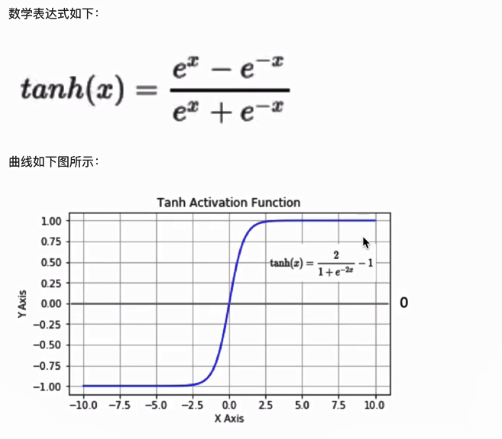③relu（最常用）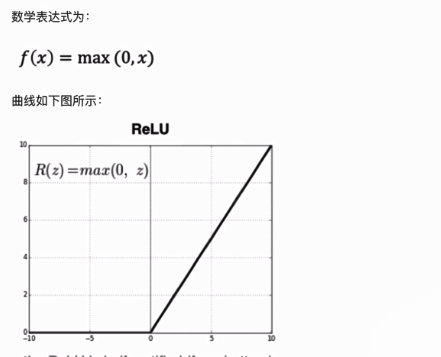为什么引入Relu呢？     第一，采用sigmoid等函数，反向传播求误差梯度时，求导计算量很大，而Relu求导非常容易。     第二，对于深层网络，sigmoid函数反向传播时，很容易就会出现梯度消失的情况（在sigmoid接近饱和区时，变换太缓慢，导数趋于0），从而无法完成深层网络的训练。     第三，Relu会使一部分神经元的输出为0，这样就造成了网络的稀疏性，并且减少了参数的相互依存关系，缓解了过拟合问题的发生（以及一些人的生物解释balabala）。
④Leakrelu(针对relu会导致神经元死亡现象的改进)
小于0时不为0，而是接近于0，当使用relu效果不好时，可以试试看leakrelu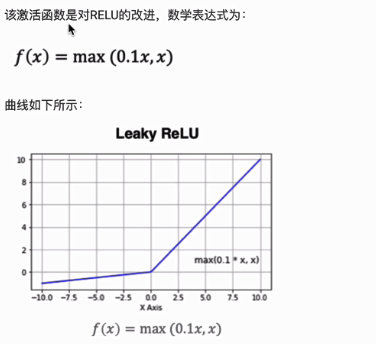⑤softmax
用于多分类过程中，是二分类函数sigmod在多分类上的推广
目的：将多分类的结果以概率的形式展现出来（当前神经元输出的结果比上所有神经元输出的结果）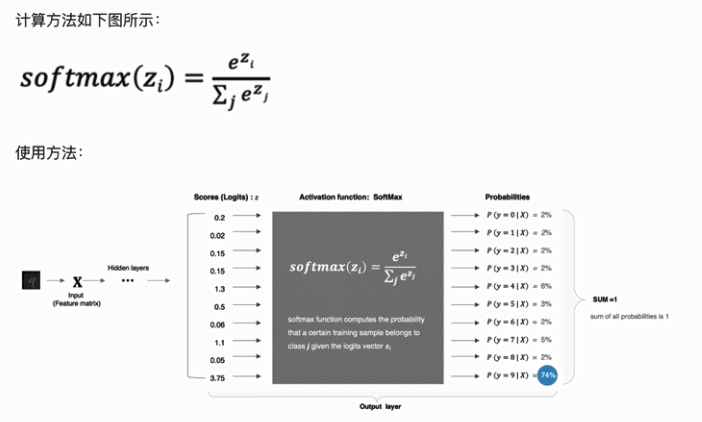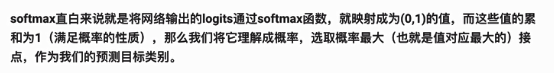⑥其他激活函数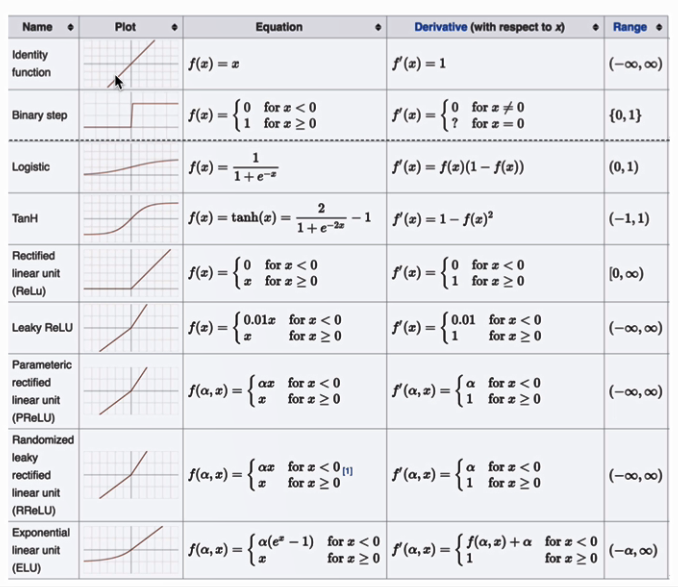四、激活函数如何选择


展开全文• 6、过拟合和欠拟合 欠拟合：模型不能很好的拟合分类 过拟合：模型对分类拟合的过于好 欠拟合的解决方法： 增加输入特征项 增加网络参数 减少正则化参数 过拟合的解决方法： 数据清洗 增大训练集 ...tensorflow
• 过拟合、欠拟合及其解决方案 训练误差和泛化误差 过拟合和欠拟合的概念 模型复杂度和误差之间的关系 解决过拟合的方案 梯度消失及梯度爆炸 循环神经网络进阶 GRU LSTM 深度神经网络 过拟合、欠拟合及其解决方案 训练...
• 文章目录1 过拟合、欠拟合及其解决方案1.1 模型选择、过拟合和欠拟合1.2 过拟合和欠拟合1.3 权重衰减1.4 丢弃法2 梯度消失、梯度爆炸2.1 梯度消失和梯度爆炸2.2 考虑环境因素3 循环神经网络进阶3.1 GRU3.2 LSTM3.3 ...
• 过拟合、欠拟合 过拟合、欠拟合的概念 权重衰减 丢弃法 训练误差与泛化误差 训练误差（training error）和泛化误差（generalization error）。通俗来讲，前者指模型在训练数据集上表现出的误差，后者指模型在任意一...
• 欠拟合和过拟合 1.1训练误差与泛化误差 在解释上述现象之前，我们需要区分训练误差（training error）和泛化误差（generalization error）。通俗来讲，前者指模型在训练数据集上表现出的误差，后者指模型在任意一个...
•深度学习 python 机器学习 人工智能 tensorflow
• 过拟合、欠拟合及解决方案知识点总结 区分两种误差 训练误差为训练数据集（training data）上的误差; 泛化误差为模型在任意一个测试数据样本上表现的误差的期望（常通过测试数据（test data）集上的误差来近似）。...
• 过拟合、欠拟合及其解决方案 过拟合和欠拟合 一类是模型无法得到较低的训练误差，我们将这一现象称作欠拟合（underfitting）； 另一类是模型的训练误差远小于它在测试数据集上的误差，我们称该现象为过拟合...
• 过拟合欠拟合及其解决方案 训练误差和泛化误差 训练误差：模型在训练数据集上表现出的误差 泛化误差：模型在任意一个测试数据样本上表现出的误差的期望，并常常通过测试数据集上的误差来近似。 机器学习模型应关注...
• 转载，原文地址：深度学习...训练一开始，模型通常会欠拟合，所以会对模型进行优化，等训练到一定程度后，就需要解决过拟合的问题了。 一、模型训练拟合的分类和表现 如何判断过拟合呢？我们在训练的时候会定义训练误
• 但是，神经网络在训练样本中表现得过于优越，导致在验证数据集以及测试数据集中表现不佳，这种情况称为过拟合。过拟合常常在模型学习能力过强的情况中出现，此时的模型学习能力太强，以至于将训练集单...数据增强 正则化
• 过拟合、欠拟合及解决方案在之前自己已经使用较多较熟练，故跳过。 梯度消失、梯度爆炸 深度模型有关数值稳定性的典型问题是消失（vanishing）和爆炸（explosion）。 当神经网络的层数较多时，模型的数值稳定性容易...
• 一开始模型往往是欠拟合的，需要神经网络优化权重参数 1.欠拟合 欠拟合现象 模型没有很好地捕捉到数据特征，不是很好的拟合 模型欠拟合 产生欠拟合的原因 数据特征项不够(ML) 训练时间不够(DL) 模型过于简单(DL...深度学习
• 【一】过拟合、欠拟合及其解决方案 过拟合 模型的训练误差远小于它在测试数据集上的误差，我们称该现象为过拟合（overfitting）。 欠拟合 当模型无法得到较低的训练误差时，我们将这一现象称作欠拟合（underfitting...
• 一、过拟合和欠拟合 训练误差和测试误差都大，欠拟合 underfitting。模型复杂度不够。 训练误差小于测试误差，过拟合 overfitting。 影响因素之一：训练数据集大小 影响欠拟合和过拟合的另一个重要因素是训练数据集...
• 一、过拟合、欠拟合及其解决方案 ·模型选择、过拟合和欠拟合 训练误差(training error)：模型在训练数据集上表现出来的误差 泛化误差(generalization error)：模型在任意一个测试样本上表现出的误差的期望，并常常...
• 一过拟合、欠拟合及其解决方案 1.1 欠拟合与过拟合 1.2 过拟合解决方案 1.3 欠拟合解决方案 二 梯度消失、梯度爆炸 2.1 梯度消失 2.2 梯度爆炸 三 循环神经网络进阶 3.1 循环神经网络的主要结构 3.2 ...
• 1.过拟合欠拟合及其解决方案 一类是模型无法得到较低的训练误差，我们将这一现象称作欠拟合（underfitting）。 模型的训练误差远小于它在测试数据集上的误差，我们称该现象为过拟合（overfitting）。 在实践中，我们...
• 循环神经网络进阶过拟合欠拟合及其解决方案模型选择、过拟合和欠拟合模型选择验证数据集K折交叉验证过拟合和欠拟合模型复杂度训练集大小权重衰减L2L_2L2​范数正则化丢弃法 过拟合欠拟合及其解决方案 模型选择、过...python 人工智能 深度学习
• 之前学的神经网络的原理、奇技淫巧什么的都忘的差不多了，正好这次有任务，顺道把之前的一些没理解透的问题重新理一理： 神经网络现在有很多类型了，每一种类型都是在之前的基础上发现问题并解决问题的过程，目前...梯度消失 梯度爆炸...